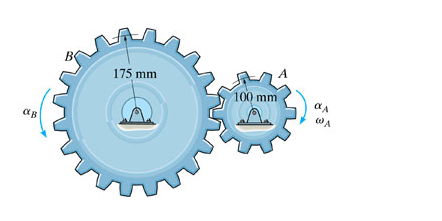# Problem: A motor gives gear A an angular acceleration of αA=(2+0.006θ2)rad/s2, where θ is in radians. If this gear is initially turning at ωA=15 rad/s, determine the angular velocity of gear B after A undergoes an angular displacement of 10 rev.Express your answer using three significant figures. Enter positive value if the angular velocity is counterclockwise and negative value if the angular velocity is clockwise.

###### FREE Expert Solution

We have angular acceleration, angular velocity, and angular displacement. The angular acceleration is raised to a power of θ. Thus, we know this is a rotational kinematics problem with calculus.

98% (186 ratings)###### Problem Details

A motor gives gear A an angular acceleration of αA=(2+0.006θ2)rad/s2, where θ is in radians. If this gear is initially turning at ωA=15 rad/s, determine the angular velocity of gear B after A undergoes an angular displacement of 10 rev.Express your answer using three significant figures. Enter positive value if the angular velocity is counterclockwise and negative value if the angular velocity is clockwise.